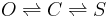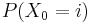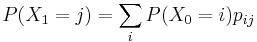# Bayesian Filtering

First we discuss Homework S.

## Bayesian Filtering Introduction

Here is the problem -- there is an open state (O) and closed state (C) and a stuck (inactivated) state (S). Transitions between these states happen like this$O \rightleftharpoons C \rightleftharpoons S$

Transitions between O and S never happen (i.e. the probability is negligible) without C being an intermediary state. The observed current is zero in C and S and one in O. And there is noise. Transitions between C and S are invisible because there is no change in current. We want to estimate the transition probabilities. (The probabilities will depend upon the time step, but say the time step is fixed).

First generate some data which looks like.

The transition probabilities for this plot are

Probability(Stay in Open) = 0.95
Probability(Open --> Closed) = 0.05
Probability(Open --> Stuck/Inactive) = 0.00

Probability(Closed --> Open) = 0.10
Probability(Stay in Closed) = 0.85
Probability(Closed --> Stuck) = 0.05

Probability(Stuck --> Open) = 0.00
Probability(Stuck -- Closed) = 0.003
Probability(Stay in Stuck) = 0.997


There are nine parameters here, but there is a constraint that each block add to 1, so we only have 6 parameter to estimate. Moreover we might know that transition between open and stuck never happen, so there would only be 4 parameters to estimate.

Thus the likelihood function is a function from four parameters to one value (likelihood). Too many to plot. Let's say we know the probabilities of going back and forth between open and closed. Thus we need only know the probabilities of going back and forth between closed and stuck (the invisible transitions).

We are going to compute the likelihood as we vary

P(Stuck --> Closed) from 0.001 to 0.010
P(Closed --> Stuck) from 0.01 to 0.12


Actually, the way I wrote the program it computes

P(Stuck --> Closed) from 0.001 to 0.010
P(Stay in Closed) from 0.01 to 0.12


The two are equivalent because P(Closed --> Open) is correctly known and the three "from Closed" probabilities add to 1.

Here are what it looks like:

And with two different seeds:

I was concerned about the first plot, until I plotted the next two. Then I realized that the algorithm tries to estimate the probabilities of relatively rare events based on a limited amount of data (5000 data points). The performance may not look great at first sight, but consider how subtle are the inferences that it is trying to make. The estimates are reasonably close ... at least within the ballpark. It would look better zoomed out. With a lot more data, one can expect the estimates to be much better.

The purpose of the lecture is to present the Bayesian filtering algorithm.

## Prerequisites

• You need to know (or guess) initial probabilities for each state. For example say we assume with certainty that the channel starts open. Then P(X0 is Open) = 1, P(X0 is Closed) = 0, P(X) is Stuck) = 0.
• You need guesses for the transition probabilities: p_ij is the probability of going to state j, assuming you start in state i. The likelihood function of interest is a function of these transition probabilities. You calculate the value of this function at your guesses, then tweak your guesses to maximize likelihood.
• You need to know (or guess) the probability density function for the data given that you are in each state. For example, in the code, densities are Gaussian, the means are (1,0,0) for (open, closed, stuck) and the standard deviation for all is 0.01. In this case the means for open and closed/stuck are separated by 100 standard deviations -- you assume essentially no measurement noise.
• You need the data.

## Step One: Start with Initial Probabilities for State And Make a Prediction for Next Time Step

Initial Probabilities$P(X_0 = i)$

Predicted Probabilities$P(X_1 = j) = \sum_i P(X_0 = i) p_{ij}$

For example, the predicted probability that you are in CLOSED after the first time step (but before more information is gathered) is

• Probability that you start OPEN times probability that you transition from OPEN to CLOSED
• PLUS Probability that you start CLOSED times probability that you stay CLOSED
• PLUS Probability that you start STUCK times probability you transition from STUCK TO CLOSED

Same formula for the other states (OPEN AND STUCK).

## Step Two: Collect data, and evaluate probability density of the data

You are computing P(data|state) for each value of state and the one data point.

## Step Three: Weight the densities above by the prior probabilities of being in each state

You are computing, for each state, P(data|state)*P(state), where P(data|state) was computed in step 2, and P(state) was computed in step 1.

## Step Four: Sum the weighted densities over the state

This sum is the marginal likelihood. As this step is repeated, the likelihood is the product of all marginal likelihoods. (Actually the log-likelihood is usually computed as the sum of the logs of the marginal likelihoods).

## Step Five: Compute the Posterior Probability Mass Function for State Given the Measurement

This is done by normalizing (dividing) the weighted densities by the marginal likelihood: P(data|state)*P(state)/MarginalLikelihood. This is Bayes Rule giving a new Probability Mass Function for State (i.e. its a function of state). This now replaces the initial Probability Mass Function.

## Repeat Steps One through Five until the data is exhausted

Use the Posterior Mass Function instead of the Initial Probability Mass Function.

## Using the Bayesian Filtering Inspector Code

BF_Inspector


The boxes below are the program's output, broken up to allow for explanation.

Initial PMF
P(Open  ) = 1
P(Closed) = 0
P(Stuck ) = 0


The above is the initial Probability Mass Function for the State, specified as parameters in the code.

-------------Step 1-------------
Prediction PMF for step 1 Conditioned on Previous 0 Measurements
P(Open  ) = 0.95
P(Closed) = 0.05
P(Stuck ) = 4.1633e-17


The inspector predicts probabilistically what will happen to the model. Because the initial state is open with certainty these values are transition probabilities from open. The non-zero P(Stuck ) is numerical error -- P(Stuck ) is not specified but rather calculated by subtracting the first two probabilities from 1. Floating point arithmetic is prone to such errors.

-------------Step 2-------------
Tell me the data point:


Now the program asks you for first data point. Let say we measure zero (what we would expect if the channel is Closed or Stuck). Type 0 and press return:

-------------Step 2-------------
Tell me the data point: 0
Probability Density of Data given State (Evaluate Bell Curve Funct)
P(Data|Open  ) = 0
P(Data|Closed) = 15.9155
P(Data|Stuck ) = 15.9155


From the measurement, we can be sure we are not in the open state (only because the noise is very low). But the measurement tells us nothing to distinguish between Closed and Stuck. In the next step, we start to combine the prior information to help us distinguish between these two states.

-------------Step 3-------------
Conditional Probability Weighted by the Prior (Prediction) PMF
P(Data|Open  )*P(Open  ) = 0
P(Data|Closed)*P(Closed) = 0.79577
P(Data|Stuck )*P(Stuck ) = 6.6262e-16


Only one of these is numerically non-zero. And that makes sense. We were sure that we were in the Open state, then the measurement told us we were no longer in the open state, so the closed state is the only place we can be.

-------------Step 4-------------
The Marginal Likelihood is the sum of the Weighted Conditional Probs
Marginal likelihood 0.79577
Running sum of logs of marginal likelihoods -0.22844


The conditional probability weighted by prior, P(data|state)*P(state), tells us how likely it is that we are in the given state AND we observe the given data point. Add them up across state and you get how likely it is that we observe the given data point (regardless of state). This sum is the marginal likelihood for the measurement of the data point. Multiply them (or add their logs) for all data points and you get the (log-) likelihood of interest.

-------------Step 5-------------
The updated state PMF is the Weighted Conditional Probs normalized
by the marginal measurement likelihood (Bayes Rule)
Posterior PMF conditioned on all prior measurements
P(Open  ) = 0
P(Closed) = 1
P(Stuck ) = 8.3267e-16


All we have done is normalize to get a PMF. Now the probabilities add to 1, forgetting numerical artifacts. Here we are certain to be in the closed state.

Now we repeat.

%%%%%%%%%%%% Data Point 2 %%%%%%%%%%%%
-------------Step 1-------------
Prediction PMF for step 2 Conditioned on Previous 1 Measurements
P(Open  ) = 0.1
P(Closed) = 0.85
P(Stuck ) = 0.05
-------------Step 2-------------
Tell me the data point:


Repeating the prediction step, these are the transition probabilities from the Closed state. If another measurement of zero is taken the state will no longer be known with certainty. Nevertheless, the PMF will provide the statistically optimal knowledge of the state. Try another measurement of 0.

## Homework

Homework T.1: Repeat data points of zero (by entering them in to BF_Inspector). Data points of zero are expected if the State is either Closed or Stuck. What happens to the probabilities of closed and stuck in the Posterior PMF as the number of zero data points increases? What happens to the prediction PMF as the number of zero data points increases? What happens if a data point = 1 is thrown in?

Homework T.2: What happens if a data point of 0.5 is thrown in? Recall the standard deviation of the noise (INoiseLevel = 0.01). How many standard deviations away from the mean of 0 or 1 is 0.5? What happens to the marginal likelihood? What happens to the log-likelihood sum? How much does the log-likelihood sum change after more measurements of 0 and 1? What happens to the posterior PMF after a measurement of 0.5? Do all the probabilities get small? How do you explain your last answer?

Homework T.3: Compare the P(Data|Open), P(Data|Closed) and P(Data|Stuck) for a measurement of 0.5. Based on these values, does a measurement of 0.5 tell you anything about the State? Repeat data points of measurements of 0.5. What happens to the Posterior PMF. Does your answer depend upon the initial Prediction PMF (start entering measurements of 0.5 after a 1 (Open), then after 1 then a 0 (Closed), then after a lot of 0's (Stuck)). Why does probability mass concentrate on one state, regardless?

Homework T.4: Edit the code for BF_Inspector.m so that the INoiseLevel (the standard deviation of the measurement noise) is 1.0 (instead of 0.01). Redo Problem T.2. Note differences.

Homework T.5: With a large noise level (1.0) does a measurement of 1 lead to certainty that state is open? How can you become more certain of an Open state? What happens when you repeat measurements of 1? How about repeat measurements of 0.